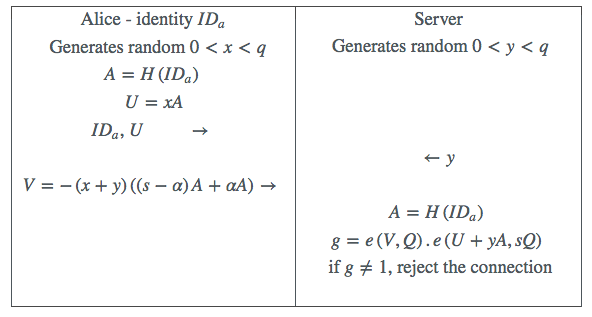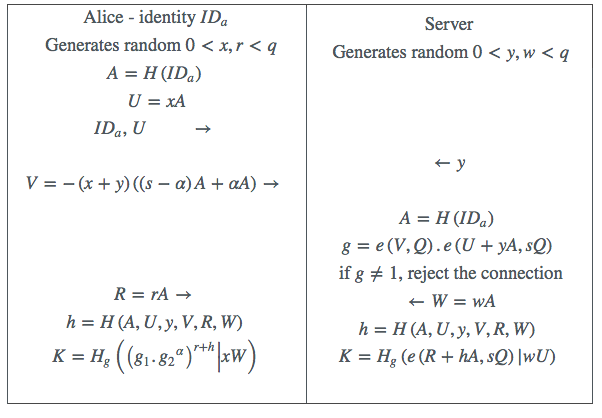# M-Pin Full Technology (Version 3.1)

##### Dr Michael Scott

M-Pin is a two-factor authentication protocol which has been proposed as an alternative to Username/Password, which works in conjunction with SSL/TLS. Here we derive a more complex M-Pin derivative called M-Pin-Full which also supplants the functionality of SSL/TLS.

### 1 Introduction

M-Pin is a zero-knowledge authentication protocol which authenticates a client to a server. Its unique feature is that it allows a short PIN number to be extracted from the client secret to create a token+PIN combination, facilitating two factor authentication. The idea can easily be extended to support multifactor authentication.

A strong client-server protocol should (a) authenticate the client to the server, (b) authenticate the server to the client, and (c) should result in a negotiated encryption key with which subsequent communications can be encrypted. The standard method of implementation uses a Username/Password mechanism to authenticate the client to the server, and the well known TLS/SSL protocol to authenticate the server to the client and to establish the encryption key. The weakest link here is the Username/Password mechanism which is widely regarded as being broken. SSL itself, to a lesser extent, has been weakened by intensive scrutiny which has revealed some exploitable vulnerabilities. To replace Username/Password, multi-factor authentication is the most often touted solution. Of all the possible form-factors the simple ATM-like combination of a token and a PIN number is the most user-familiar and user-friendly.

Here we extend the M-Pin technology solution to also replace the SSL functionality. Recall that M-Pin makes use of a Trusted Authority to issue client and server secrets. Using M-Pin, no client secrets, or values derived from client secrets, are stored on the server. The reader is encouraged to read the M-Pin paper before continuing with this white paper.

### 2 M-Pin

Here we recall the original M-Pin protocol. Alice is proving to a server that she is in possession of a valid secret, while revealing nothing about it, using a Zero-Knowledge Proof protocol.

A Trusted Authority (TA) possesses a unique secret value$s$ associated with its support for a particular server. That server is issued with a secret $sQ$, which represents a fixed point$Q$ on a special elliptic curve multiplied by the TA secret $s$. Alice, whose identity string is $I{D}_{a}$, has this identity hashed and mapped to a point$A$ on the same curve (albeit a different group of the same order $q$), and is issued with the secret $sA$. Alice chooses a PIN number $\alpha$, and extracts this from her secret to create her token $\left(s-\alpha \right)A$. The protocol then proceeds as follows.Table 1: M-Pin

This all works thanks to the pairing function$e\left(.,.\right)$ and its remarkable bilinearity property $e\left(aP,Q\right)=e\left(P,aQ\right)=e{\left(P,Q\right)}^{a}$.

### 3 M-Pin-Full

This more elaborate protocol not only replaces Username/Password, but replaces the functionality of SSL as well. Our starting point is the M-Pin protocol as described above. The idea is to run it first (to authenticate the client to the server), and then proceed to authenticate the server to the client via an authenticated key exchange, which also establishes the agreed key.

The first thing to note is that both the client and the server can already calculate a mutual authenticated encryption key! The client Alice can calculate it as $e\left(sA,Q\right)$, and the server as $e\left(A,sQ\right)$. Note that for a client this is a fixed value that can be precomputed. Now the TA also issues to Alice${g}_{1}=e\left(sA,Q\right)$ and ${g}_{2}=e\left(A,Q\right)$. Next Alice extracts the PIN from${g}_{1}$ by calculating ${g}_{1}={g}_{1}∕{{g}_{2}}^{\alpha }=e\left(\left(s-\alpha \right)A,Q\right)$. Both${g}_{1}$ and${g}_{2}$ can be stored on the client along with the token.

The full secret can then be reconstructed when the PIN is available as ${g}_{1}.{{g}_{2}}^{\alpha }$, which only requires a small amount of work as$\alpha$ is small.

However we must be careful to (a) protect the PIN from an active or passive attacker who has perhaps captured the token, (b) prevent a Key Compromise Impersonation (KCI) attack, and (c) achieve the property of Perfect Forward Secrecy (PFS). To support the property of PFS, the standard approach adopted here is to introduce a Diffie-Hellman component into the protocol.

This protocol requires another general hash function${H}_{g}\left(.\right)$ which serializes, and hashes its input to a 256-bit value. Both sides can then extract an AES key from this value $K$. It is left as a simple exercise for the reader to confirm that both client and server end up with the same key. Note that since the first part of the protocol is just the original M-Pin protocol, all of its features and extensions still apply. In particular Time Permits can be used as a revocation mechanism.Table 2: M-Pin-Full

Note that the transmission of$R$ from the client to the server can be done at the same time as$V$ is transmitted, and the transmission of$W$ from the server to the client can be done at the same time as$y$ is transmitted, to avoid introducing any extra flows into the protocol.

### 4 Security – Informal

Our main concern is with an attacker who has obtained a client token and is in a position to launch an active attack on the client’s attempted authentication in order to determine their PIN.

For example if a client were simply to go ahead and start encrypting using the shared key $e\left(sA,Q\right)$, then an attacker who knew the token$\left(s-\alpha \right)A$ could exhaustively try adding to the token every possible multiple of$A$ to create$X$ until they hit on the right PIN, in which case$X=sA$ and the key$e\left(X,Q\right)$ would decrypt the ciphertext to something sensible. To prevent this we actually use as the key $e\left(rsA,Q\right)$, and now an attackers knowledge of the token cannot be used to guess the key without knowing $r$.

A more subtle attack is also possible. An attacker who has captured Alice’s credentials can pretend to be a valid server to Alice by simply ignoring the initial M-Pin protocol and then also calculating the mutual key as$e\left(sA,Q\right)$ (rather than as$e\left(A,sQ\right)$ as a valid server would). However the presence of$r$ in the calculation of the key also prevents this Key Compromise Impersonation (KCI) attack.

Another type of KCI attack would arise if an attacker who had captured the server secret$sQ$ were able to use it to authenticate as a valid client. Fortunately this is not possible, as$sQ$ is in the wrong group, and therefore such an attacker will not be able to proceed beyond the first part of the protocol.

An active attacker might allow Alice to complete the first part of the protocol and then attempt to hijack the link before the calculation of the key. But observe how the value of$x$ is re-used for the calculation of the Diffie-Hellman component of the key. This binds both parts of the protocol together and effectively blocks any hijacking attempt.

### 5 Security - Formal

Here we concentrate on the security of the key exchange component of the overall protocol. Recall that by the time the first part of the protocol is completed, the client long term secret key has been reconstituted from its factors, and the client has already authenticated successfully to the server.

The basic key exchange consists of the transmission of$rA$ from the client to the server, and the calculation by the client of the partial key $e{\left(sA,Q\right)}^{r+h}$. The server can calculate the same partial key as $e\left(R+hA,sQ\right)$. The value$h$ is just a hash of all of the data exchanged between the two parties.

Next we show that this one-pass key exchange is equivalent to the one-pass variant of Wang’s IDAK key agreement protocol , section 9.5. In Wang’s protocol both partial keys are further raised to a power of $1+{h}_{s}$, where${h}_{s}$ is composed from a different ordering of the exchanged data. However$1+{h}_{s}$ is publicly known. As is well known the power of a pairing inherits all of the properties of a pairing, and therefore we choose to use the$1∕\left(1+{h}_{s}\right)$ power, which has the effect of cancelling out this term. Nevertheless Wang’s IDAK is a secure key exchange protocol, and we inherit that same property.

Wang’s protocol is proven secure in the random oracle model under the DBDH assumption, in the Bellare and Rogaway BR93 security model . However one-pass key exchange protocols cannot provide for sender KCI resistance  – for that we must assume that such an attacker is effectively blocked from progressing to the key exchange part of our protocol by the sender authentication that has already taken place.

Finally we observe that essentially the same protocol is described by Chow and Choo , and proven secure under the computational BDH (Bilinear Diffie-Hellman) assumption, in the Canetti-Krawczyk (CK) security model . Furthermore Gorantla, Boyd and Nieto [4 (section 4.3)] extend this protocol again to our one-pass setting, and provide a proof in a modified extended Canetti-Krawczyk (eCK) setting.

One minor issue is the particular variant of the BDH problem on which our security is based, in the context of our use of a type-3 pairing. In this setting the relevant BDH variant is Galbraith’s BDH-3c assumption .

### 6 Discussion

It should be pointed out that M-Pin-Full is not entirely equivalent to the SSL+M-Pin combination. The client identity is transmitted in the clear in M-Pin-Full, whereas with SSL the entire M-Pin protocol runs under cover of SSL, which therefore provides an anonymity feature. Of course it is always possible to run the M-Pin-Full protocol in conjunction with SSL. An alternative solution would be for the client to execute and complete the protocol transmitting the hash of their identity instead of the identity itself, and then to transmit their actual identity when the protocol had completed under the protection of the negotiated key. The server could then compare the hash of this identity with that transmitted earlier.

One important advantage compared to the SSL+M-Pin combination is that any so-called phishing attack will be ineffective against this protocol, as the phishing website will not be able to establish the mutual key $K$.

1. C. Boyd and A. Mathuria. Protocols for Authentication and Key Establishment. Springer-Verlag, 2003.

2. S. Chatterjee and A. Menezes. On cryptographic protocols employing asymetric pairings. Discrete Applied Mathematics, 159(13):1311–1322, 2011.

3. S. Chow and K. Choo. Strongly-secure identity-based key agreement and anonymous extension. In Information Security, pages 203–220. Springer-Verlag, 2007. http://eprint.iacr.org/2007/018.

4. M. Gorantla, C. Boyd, and J. Nieto. ID-based one-pass authenticated key agreement. In AISC08, pages 38 – 46. Australian Computer Society, 2008.

5. Y. Wang. Efficient identity-based and authenticated key agreement protocol. Cryptology ePrint Archive, Report 2005/108, 2005. http://eprint.iacr.org/2005/108.